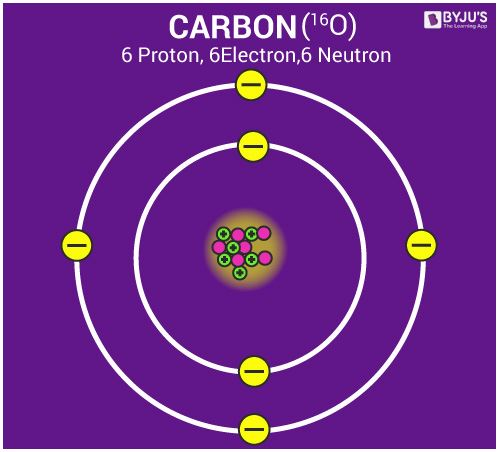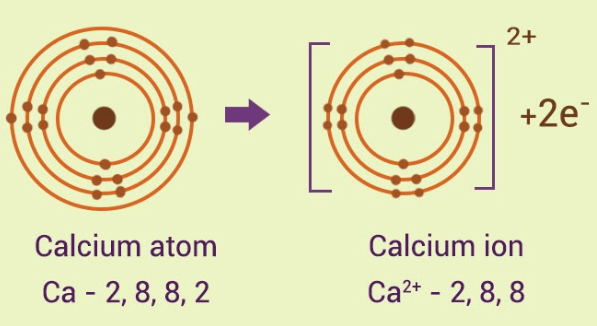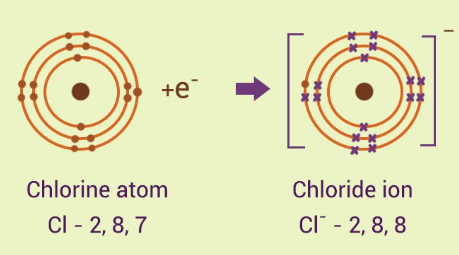Atoms and molecules are responsible for forming tiny sand particles, gargantuan black holes and everything in between. The atom is the most fundamental unit of matter, making up everything that we see around us. It is extremely small, measuring in at less than 0.1 to 0.5 nanometers.

## Laws of Chemical Combination

### Chemical Reactions

• In a chemical reaction, two or more molecules interact to produce new compounds and are called reactants, whereas the newly formed compounds are called products.
• In a chemical reaction, a chemical change must occur, which is generally observed with physical changes like precipitation, heat production, colour change, etc.

### Law of conservation of mass

• According to the law of conservation of mass, matter can neither be created nor destroyed in a chemical reaction. It remains conserved.
• Mass of reactants will be equal to the mass of products.

### Law of constant proportions

• A pure chemical compound contains the same elements combined together in a fixed proportion by mass is given by the law of definite proportions.
• For e.g., If we take water from a river or from an ocean, both have oxygen and hydrogen in the same proportion.

### Atoms

An atom is the defining structure of an element, which cannot be broken by any chemical means.

The atomic symbol has three parts:-

• The symbol X: the usual element symbol
• The atomic number A: equal to the number of protons
• The mass number Z: equal to the total number of protons and neutrons in an element.

### Dalton’s Atomic Theory

1. The matter is made up of indivisible particles known as atoms.
2. The properties of all the atoms of a given element are the same, including mass. This can also be stated as all the atoms of an element have identical mass and chemical properties; atoms of different elements have different masses and chemical properties.
3. Atoms of different elements combine in fixed ratios to form compounds.
4. Atoms are neither created nor destroyed. The formation of new products (compounds) results from the rearrangement of existing atoms (reactants) in a chemical reaction.
5. The relative number and kinds of atoms are constant in a given compound.

To know more about Laws of Chemical Combination, visit here.

## Atomic Mass

### Atomic mass and atomic mass unit

• Atomic mass is the total of the masses of the electrons, neutrons, and protons in an atom, or in a group of atoms, the average mass.
• Mass of an atomic particle is called the atomic mass.
• This is commonly expressed as per the international agreement in terms of a unified atomic mass unit (AMU).
• It can be best defined as 1/12 of the mass of a carbon-12 atom in its ground state.

To know more about Atomic Mass, visit here.

### Molecular mass

Molecular mass of an element is defined as the sum of the masses of the elements present in the molecule.

• Molecular mass is obtained by multiplying the atomic mass of an element with the number of atoms in the molecule and then adding the masses of all the elements in the molecule.

To know more about Molecular mass, visit here.

## Mole Concept

### Mole concept & Avogadro Number

• In a substance, the amount of entities present for e.g. atoms, molecules, ions, is defined as a mole. A mole of any substance is 6.022×1023 molecules.
• Mole concept is one of the most convenient ways of expressing the amount of reactants and product in the reaction.

The value of Avogadro’s number is approximately 6.022×1023. The definition of Avogadro’s number is that it tells us the number of particles in 1 mole (or mol) of a substance. These particles could be electrons or molecules or atoms.

To know more about Mole Concept, visit here.

## Molar Mass

### Molar mass

A substance is something which has mass and occupies space. The molar mass/molecular weight is actually the sum of the total mass in grams of the atoms present to make up a molecule per mole. The unit of molar mass is grams/mole.

To know more about Molar Mass, visit here.

## Atomic Valency

### Molecules and Atomicity

A molecule is defined as the smallest unit of a compound that contains the chemical properties of the compound.

• The atomicity of an element is the number of atoms in one molecule of the element.
• For e.g:- Hydrogen, nitrogen, oxygen, chlorine, iodine, bromine all have two atoms in each of their molecules. So, the atomicity of hydrogen, nitrogen, oxygen, chlorine, iodine, bromine is two each.

### Structure of atom

• Atom is made of three particles; electron, proton and neutron.
• The centre of the atom is called the nucleus. The nucleus of an atom contains the whole mass of an atom.
• Electrons in an atom are arranged in shells/orbitals.### Valency

Valence electrons are those electrons which are present in the outermost orbit of the atom.

• The capacity of an atom to lose, gain or share valence electrons in order to complete its octet determines the valency of the atom.

To know more about Valency, visit here.

## Writing Chemical Formulae

### Compounds

• When two or more elements chemically combine in a fixed ratio by mass, the obtained product is known as a compound.
• Compounds are substances consisting of two or more different types of elements in a fixed ratio of its atoms.

### Ions

• An ion is defined as an atom or molecule which has gained or lost one or more of its valence electrons, giving it a net positive or negative charge.
• A negatively charged particle is called an anion, and a positively charged particle is called a cation.

### Ionic compounds: chemical formula

• Ionic compounds are chemical compounds in which ions are held together by specialised bonds called ionic bonds.
• An Ionic compound always contains an equal amount of positive and negative charge.
• For example: In Calcium chloride, the ionic bond is formed by oppositely charged calcium and chloride ions.
Calcium atom loses 2 electrons and attains the electronic configuration of the nearest noble gas (Ar). By doing so, it gains a net charge of +2.The two Chlorine atoms take one electron each, thus gaining a charge of -1 (each) and attain the electronic configuration of the nearest noble gas (Ar).To know more about Writing Chemical Formulae, visit here.

Explore more questions, concepts and tips on atoms and molecules Class 9 notes by registering at BYJU’S.

## Frequently Asked Questions on CBSE Class 9 Science Notes Chapter 3: Atoms and Molecules

### What is difference between atoms and molecules?

Atoms refer to the mos basic or smallest unit of a chemical element. Whereas, molecules are composed of 2 or more atoms held together.

### Who was Dalton?

John Dalton was an English chemist, physicist and he was best known for his Atomic Theory.

### What is a mole?

Mole is a standard unit for measuring the amount of any substance.

#### 1 Comment

1. Mohit Lokhande

It is the best website for maths , science